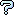All about flooble | fun stuff | Get a free chatterbox | Free JavaScript | Avatarsperplexus dot infoPowerful Couple (Posted on 2011-03-16)(A) For a base ten positive integer P drawn at random between 10 and 99 inclusively, determine the probability that the first two digits (reading left to right) in the base ten expansion of 2P is equal to P-1.

(B) For a base ten positive integer P drawn at random between 10 and 99 inclusively, determine the probability that the first two digits (reading left to right) in the base ten expansion of 6P is equal to P-1.

 No Solution Yet Submitted by K Sengupta No RatingComments: ( Back to comment list | You must be logged in to post comments.)re: exploration turned up something strange| Comment 3 of 6 |(In reply to exploration turned up something strange by Jer)

I'm not sure what you are referring to. To begin with I don't know what a non-integral solution would be, as the first two digits would form an integer and so could be 1 less than some other integer.

Do you mean something like 2^13.55075 = 12000.026738460294, where the integer part of P equals 1 more than the integer formed from the first two digits of 2^P?

Edited on March 16, 2011, 3:42 pm
 Posted by Charlie on 2011-03-16 15:41:49Please log in:

 Search: Search body:
Forums (5)